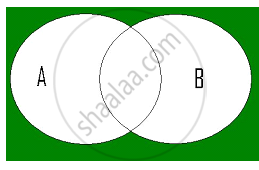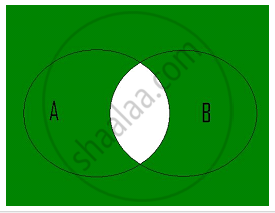Share

# Complement of a Set

#### description

• Properties of Complement Sets

#### notes

Let U be the universal set and A a subset of U. Then the complement of A is the set of all elements of U which are not the elements of A. Symbolically, we write A′ to denote  the complement of A with respect to U.
Thus, A′ = {x : x ∈ U and x ∉ A }. Obviously A′ = U – A
We note that the complement of a set A can be looked upon, alternatively, as the difference between a universal set U and the set A.
Example-  Let U =  {1, 2, 3, 4, 5, 6, 7, 8, 9, 10} and A = {1, 3, 5, 7, 9}. Find A′.
Solution-  A′ = {2, 4, 6, 8,10}

De Morgan's Law:

1)The complement of the union of two sets is the intersection of their complements.
(A ∪ B)′= A′ ∩ B′2) The complement of the intersection of two sets is the union of their complements.
(A ∩ B)′= A′ ∪ B′Some Properties of Complement Sets

1) Complement laws:
i) A ∪ A′  = U
ii) A ∩ A′ = Ø
2) De Morgan’s law:
i) (A ∪ B)´  = A′ ∩ B′
ii) (A ∩ B)′ = A′ ∪ B′
3) Law of double complementation : (A′)′ = A
4) Laws of empty set and universal set  Ø′= U and U′ = Ø.

### Shaalaa.com

Complement of a Set [00:04:57]
S
0%